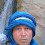## Pages

### How to create a ROC curve in R

ROC stands for Receiver Operating Characteristics, and it is used to evaluate the prediction accuracy of a classifier model. ROC curve is a metric describing the trade-off between the sensitivity (true positive rate, TPR) and specificity (false positive rate, FPR) of a prediction in all probability cutoffs (thresholds). It can be used for binary and multi-class classification accuracy checking. To evaluate the ROC in multi-class prediction, we create binary classes by mapping each class against the other classes.

In this tutorial, you'll learn how to check the ROC curve in R. We use 'ROCR' package in this tutorial. Since ROC is created by the TPR and FPR value, here I'll mention the formula of both metrics. Please refer my previous post about the confusion matrix to understand below metrics.

#### FPR = FP / (FP + TN)

First, we'll generate sample dataset and build a classifier with a logistic regression model, then predict the test data. Based on prediction data we'll create a ROC curve and find out some other metrics.

`library(ROCR)`
`  `
```df = data.frame(a=sample(1:25,400,replace = T),
b=runif(400)*3,
c=sample(1:10,400,replace = T))

df = cbind(df,type=ifelse((df\$a+df\$b+df\$c)>=20, "high", "low")) ```
` `
```index = sample(1:nrow(df), size = .80 * nrow(df))
train = df[index, ]
test = df[-index, ]

model = glm(type~a+b,data=train,

pred = predict(model,test,type="response")```
` `

Performance check

Next, we'll use a 'prediction' and 'performance' functions of a 'ROCR' package to check the accuracy. Accuracy versus cutoff values plot is shown below.

```pred = prediction(pred, test\$type)
perf = performance(pred, "acc")
plot(perf)```

You can also check the other metrics with a 'performance' function and visualize them in a plot.

```perf_cost = performance(pred, "cost")
perf_err = performance(pred, "err")
perf_tpr = performance(pred, "tpr")
perf_sn_sp = performance(pred, "sens", "spec")```

`plot(perf_cost)`

We can get maximum accuracy cutoff from accuracy performance.

```max_ind = which.max(slot(perf, "y.values")[] )
acc = slot(perf, "y.values")[][max_ind]
cutoff = slot(perf, "x.values")[][max_ind]
print(c(accuracy= acc, cutoff = cutoff))```
` `
```  accuracy cutoff.347
0.9375000  0.5627766```
` `
` `

ROC curve

Next, we'll create a ROC curve.

```roc = performance(pred,"tpr","fpr")
plot(roc, colorize = T, lwd = 2)
abline(a = 0, b = 1) ```
` `

A random guess is a diagonal line and the model does not make any sense. If the curve approaches closer to the top-left corner, model performance becomes much better. Any curve under the diagonal line is worst than a random guess. We can set the cutoff threshold based on our requirement in terms of sensitivity and specificity importance.

AUC

The AUC represents the area under the ROC curve. We can evaluate the model the performance by the value of AUC. Higher than 0.5  shows a better model performance. If the curve changes to rectangle it is perfect classifier with AUC value 1.

```auc = performance(pred, measure = "auc")
print(auc@y.values)
[]
 0.9756098```

In this tutorial, we've briefly learned how to build a ROC curve and find out AUC with ROCR package. The full source code is listed below.

Source Code Listing

` `
```library(ROCR)

df = data.frame(a=sample(1:25,400,replace = T),
b=runif(400)*3,
c=sample(1:10,400,replace = T))

df = cbind(df,type=ifelse((df\$a+df\$b+df\$c)>=20, "high", "low"))

index = sample(1:nrow(df), size = .80 * nrow(df))
train = df[index, ]
test = df[-index, ]

model = glm(type~a+b,data=train,

pred = predict(model,test,type="response")
pred = prediction(pred, test\$type)
perf = performance(pred, "acc")
plot(perf)

max_ind = which.max(slot(perf, "y.values")[] )
acc = slot(perf, "y.values")[][max_ind]
cutoff = slot(perf, "x.values")[][max_ind]
print(c(accuracy= acc, cutoff = cutoff))
```
```perf_cost = performance(pred, "cost")
perf_err = performance(pred, "err")
perf_tpr = performance(pred, "tpr")
perf_sn_sp = performance(pred, "sens", "spec")

roc = performance(pred,"tpr","fpr")
plot(roc, colorize = T, lwd = 2)
abline(a = 0, b = 1)

auc = performance(pred, measure = "auc")
print(auc@y.values) ```

References:

1.for:
model = glm(type~a+b,data=train,
Error in eval(family\$initialize) : y values must be 0 <= y <= 1
>

1.I think it's because y needs to be a factor instead of a character, what I did was just change the "high" to 1 and "low" to 0 in the ifelse statement :)

2.if(!require(ROCR)){
install.packages("ROCR")
library(ROCR)
}

library(ROCR)

df = data.frame(a=sample(1:25,400,replace = T),
b=runif(400)*3,
c=sample(1:10,400,replace = T))

df\$type

\$ df = cbind(df,type=ifelse((df\$a+df\$b+df\$c)>=20, "high", "low"))

df = cbind(df,type=ifelse((df\$a+df\$b+df\$c)>=20, 1, 0))

index = sample(1:nrow(df), size = .80 * nrow(df))

index

train = df[index, ]

train

test = df[-index, ]

test

attach(df)

str(df)

df

df\$type = as.factor(df\$typ)

str(df)

model = glm(type~a+b,data=train,

pred = predict(model,test,type="response")
pred = prediction(pred, test\$type)
perf = performance(pred, "acc")
plot(perf)

max_ind = which.max(slot(perf, "y.values")[] )
acc = slot(perf, "y.values")[][max_ind]
cutoff = slot(perf, "x.values")[][max_ind]
print(c(accuracy= acc, cutoff = cutoff))

perf_cost = performance(pred, "cost")
perf_err = performance(pred, "err")
perf_tpr = performance(pred, "tpr")
perf_sn_sp = performance(pred, "sens", "spec")

roc = performance(pred,"tpr","fpr")
plot(roc, colorize = T, lwd = 2)
abline(a = 0, b = 1)

auc = performance(pred, measure = "auc")
print(auc@y.values)

3.HOW DO I GET THE ADJUSTED CUT OF POINT OF MY AUC PLEASE